Study Materials: ncert solutions

Our ncert solutions for Exercise 9.3 (Available) Class 11 maths 9. Sequences and Series - ncert solutions - Toppers Study is the best material for English Medium students cbse board and other state boards students.

Solutions ⇒ Class 11th ⇒ Mathematics ⇒ 9. Sequences and Series

# Exercise 9.3 (Available) Class 11 maths 9. Sequences and Series - ncert solutions - Toppers Study

Topper Study classes prepares ncert solutions on practical base problems and comes out with the best result that helps the students and teachers as well as tutors and so many ecademic coaching classes that they need in practical life. Our ncert solutions for Exercise 9.3 (Available) Class 11 maths 9. Sequences and Series - ncert solutions - Toppers Study is the best material for English Medium students cbse board and other state boards students.

## Exercise 9.3 (Available) Class 11 maths 9. Sequences and Series - ncert solutions - Toppers Study

CBSE board students who preparing for class 11 ncert solutions maths and Mathematics solved exercise chapter 9. Sequences and Series available and this helps in upcoming exams 2023-2024.

### You can Find Mathematics solution Class 11 Chapter 9. Sequences and Series

• All Chapter review quick revision notes for chapter 9. Sequences and Series Class 11
• NCERT Solutions And Textual questions Answers Class 11 Mathematics
• Extra NCERT Book questions Answers Class 11 Mathematics
• Importatnt key points with additional Assignment and questions bank solved.

NCERT Solutions do not only help you to cover your syllabus but also will give to textual support in exams 2023-2024 to complete exercise 9.3 maths class 11 chapter 9 in english medium. So revise and practice these all cbse study materials like class 11 maths chapter 9.3 in english ncert book. Also ensure to repractice all syllabus within time or before board exams for ncert class 11 maths ex 9.3 in english.

See all solutions for class 11 maths chapter 9 exercise 9 in english medium solved questions with answers.

### Exercise 9.3 (Available) class 11 Mathematics Chapter 9. Sequences and Series

• Exercise 9.3 (Available) Class 11 Maths 9. Sequences And Series - Ncert Solutions - Toppers Study
• Class 11 Ncert Solutions
• Solution Chapter 9. Sequences And Series Class 11
• Solutions Class 11
• Chapter 9. Sequences And Series Exercise 9.3 (Available) Class 11

## Exercise 9.3 (Available) Class 11 maths 9. Sequences and Series - ncert solutions - Toppers Study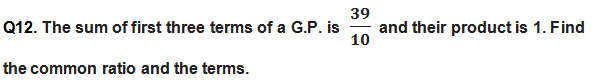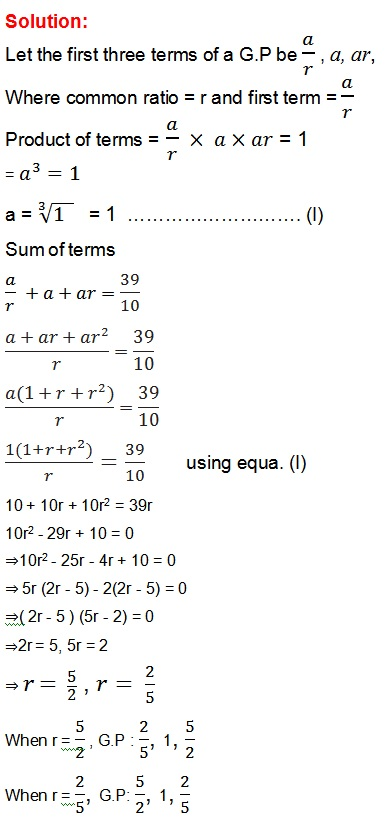Q13. How many terms of G.P. 3, 32, 33, …. are needed to give the sum 120?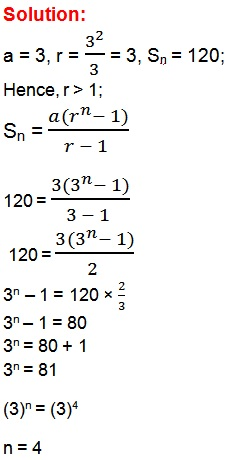Q14. The sum of first three terms of a G.P. is 16 and the sum of the next three terms is 128. Determine the first term, the common ratio and the sum to n terms of the G.P.

Solution: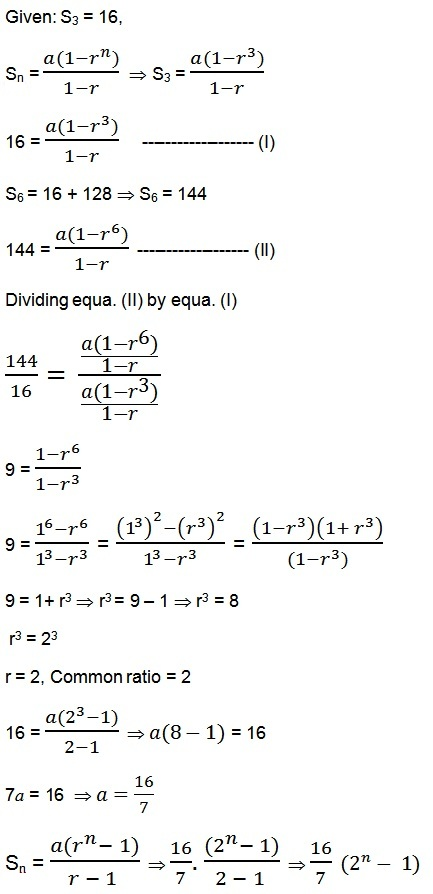Q15. Given a G.P. with a = 729 and 7th term 64, determine S7.

Solution: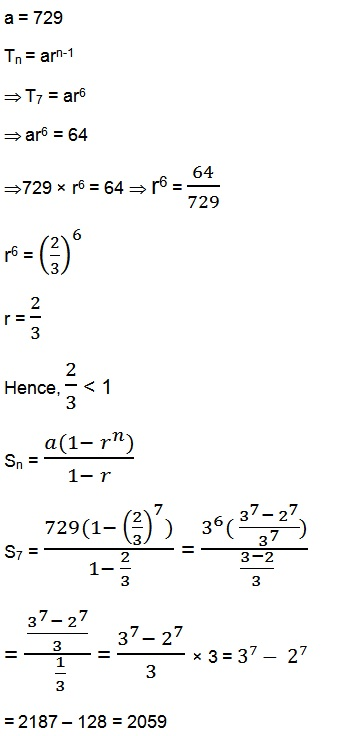Q16. Find a G.P. for which sum of the first two terms is – 4 and the fifth term is 4 times the third term.

Solution:

S2 = - 4,

T5 = 4(T3Q17. If the 4th, 10th and 16th terms of a G.P. are x, y and z, respectively. Prove that x, y, z are in G.P.

Solution:

T4 = ar3 = x ------------------ (I)

T10 = ar9 = y    ---------------(II)

T16 = ar15  = z  ---------------(III)

If T4, T10 and T16 are in G.P then x, y and z also will be in G.PQ18. Find the sum to n terms of the sequence, 8, 88, 888, 8888… .

Solution:

Let S is the sum of n terms of series;

∴ Sn  = 8 + 88 + 888 + 8888 + ………….. to the n term

= 8(1 + 11 + 111 + 1111 + ……….. )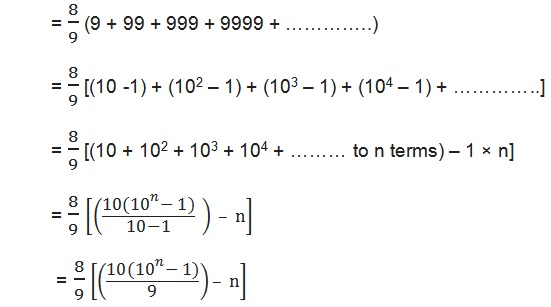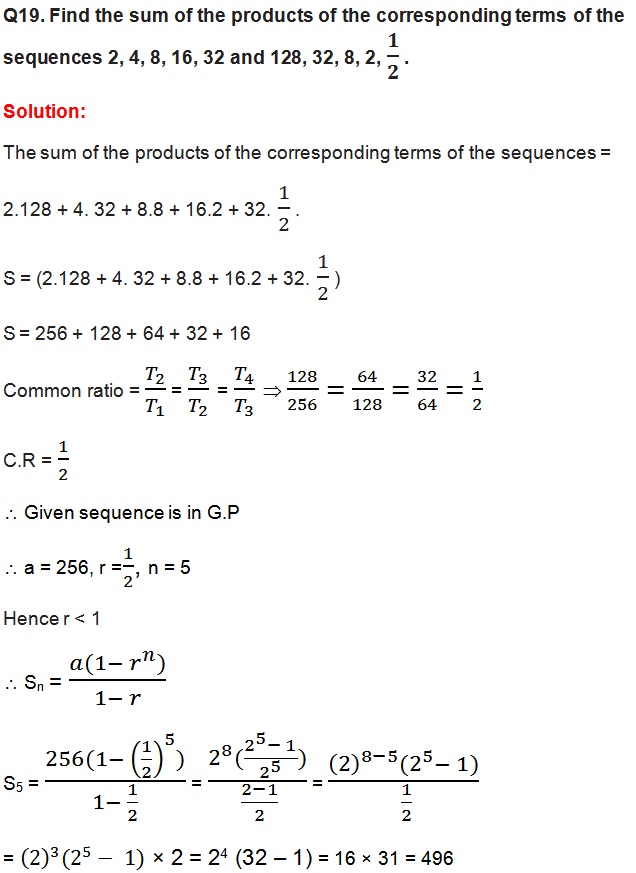Q20. Show that the products of the corresponding terms of the sequences a, ar, ar2…arn – 1 and A, AR, AR2, … ARn – 1 form a G.P, and find the common ratio.

Solution:

Product of sequence = a.A, ar.AR, ar2.AR2 ………….. arn -1.ARn -1

Common ratio :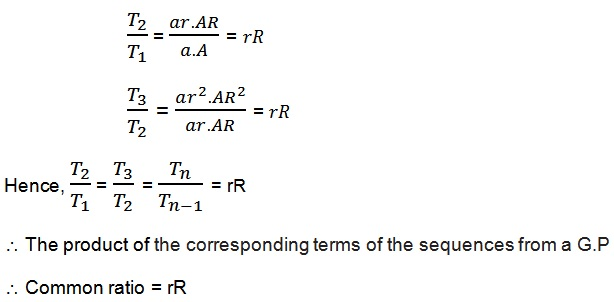Q21. Find four numbers forming a geometric progression in which the third term is greater than the first term by 9, and the second term is greater than the 4th by 18.

Solution: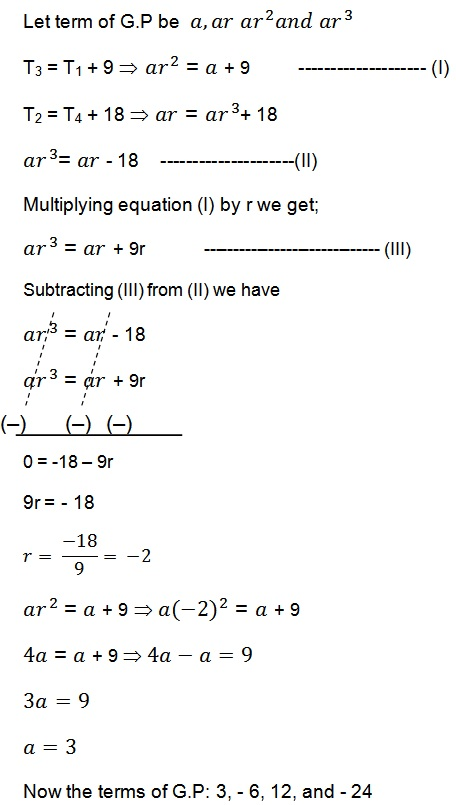Q22. If the pth, qth and rth terms of a G.P. are a, b and c, respectively. Prove that

aq – r br – p cp – q = 1.

Solution:

Let first term be A and common ratio be R.

Tp = ARp – 1  = a -------------- (I)

Tq = ARq – 1 = b -------------- (II)

Tr = ARr – 1 = c --------------- (III)

aq – r . br – p . cp – q = (ARp – 1 )q – r . (ARq – 1)r – p  . (ARr – 1)p – q

= AR(p – 1)(q – r ).AR(q – 1)(r – p) . AR(r – 1)(p – q)

= AR(p – 1)(q – r ) + (q – 1)(r – p) + (r – 1)(p – q)

= AR(pq – pr – q + r + qr – pq – r + p + pr – qr – p + q)

= (AR)0

= 1

Q23. If the first and the nth term of a G.P. are a and b, respectively, and if P is the product of n terms, prove that P2 = (ab)n.

Solution:Q24. Show that the ratio of the sum of first n terms of a G.P. to the sum of terms from (n + 1)th to (2n)th term is 1/rn.

Solution:Q25.  If a, b, c and d are in G.P. show that

(a2 + b2 + c2) (b2 + c2 + d2) = (ab + bc + cd)2.

Solution:

a, b, c, d are in G.P. Therefore,
b2 = ac …………………….... (2)
c2 = bd …………………...... (3)
It has to be proved that,

(a2 + b2 + c2) (b2 + c2 + d2) = (ab + bc – cd)2
R.H.S.
= (ab + bc + cd)2

= (ab + ad + cd)2 [Using (1)]

= [ab + d (a + c)]2

= (ab)2 + 2(ab)d(a + c) + [d(a + c)]2

= a2b2 + 2abd (a + c) + d2 (a + c)2

= a2b2  +2a2bd + 2acbd + d2(a2 + 2ac + c2)

[Using (1) and (2)]

=  a2b2  + 2a2c2 + 2b2c2 + d2a2 + 2d2b2 + d2c2  [using bc = ad and b2 = ac]

=  a2b2  + a2c2 + a2c2 + b2c2 + b2c2 + d2a2 + d2b2 + d2b2 + d2c2

=  a2b2  + a2c2 + (ac)2 + b2c2 + b2c2 + a2d2 + (bd)2 × (bd)2 + c2d2

=  a2b2  + a2c2 + (b2)2 + b2c2 + b2c2 + a2d2 + (bd)2 × (c2)2 + c2d2

=  a2b2  + a2c2 + b2 × b2 + b2c2 + c2b+ a2d2 + b2d2 + c2 × c2 + c2d2

=  a2b2  + a2c2 + a2d2 + b2 × b2 + b2c2 + b2d2 + c2b2 + c2 × c2 + c2d2

[Using (2) and (3) and rearranging terms]

= a2(b2 + c2 + d2) + b2 (b2 + c2 + d2) + c2 (b2+ c2 + d2)
= (a2 + b2 + c2) (b2 + c2 + d2) = L.H.S.
∴ L.H.S. = R.H.S.
∴ (a2 + b2 + c2) (b2 + c2 + d2) = (ab + bc – cd)2

Q26. Insert two numbers between 3 and 81 so that the resulting sequence is G.P.

Solution:

Let G1, G2 be three numbers between 3 and 81 such that 3, G1, G2, 81 is a G.P

T1 = 3

T2 = ar

T3 = ar2

T4 = ar3 = 81

3.r3 = 81

r3 =

r3 = 27For r = 3, we have

T2 = ar = 3.3 = 9

T3 = ar2 = 3.32 = 27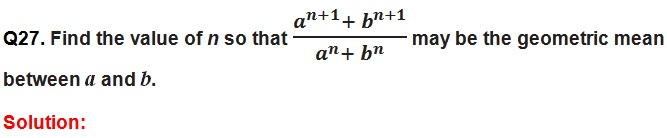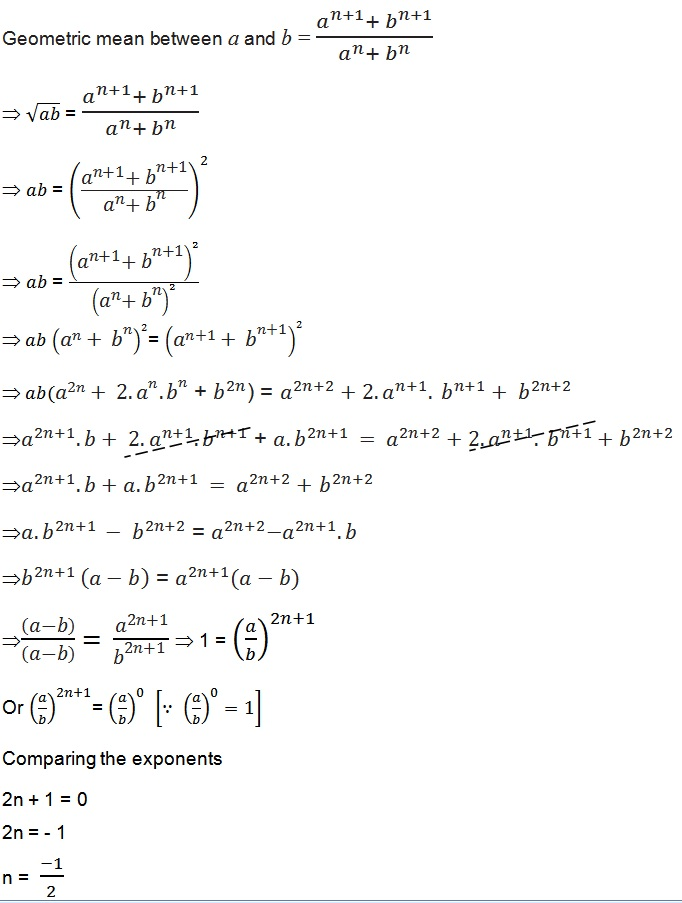Q28. The sum of two numbers is 6 times their geometric mean, show that numbers are in the ratio (3 + 2√2) : (3 − 22).

Solution:

Let the numbers be a and b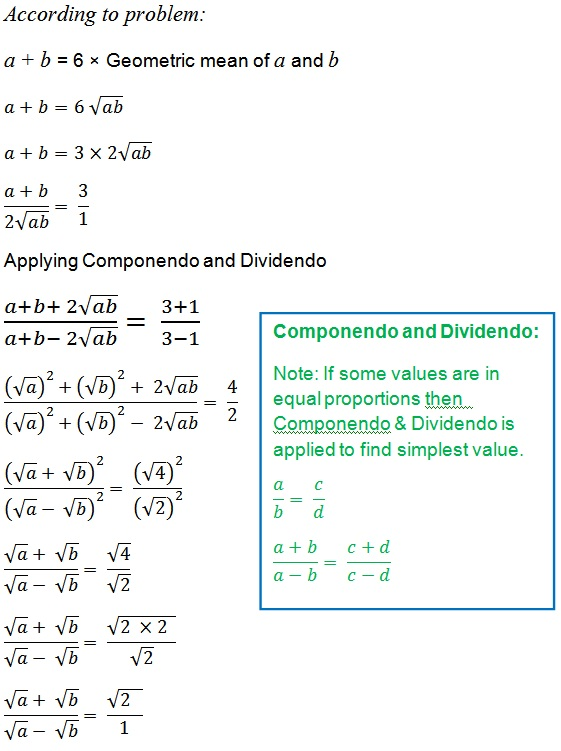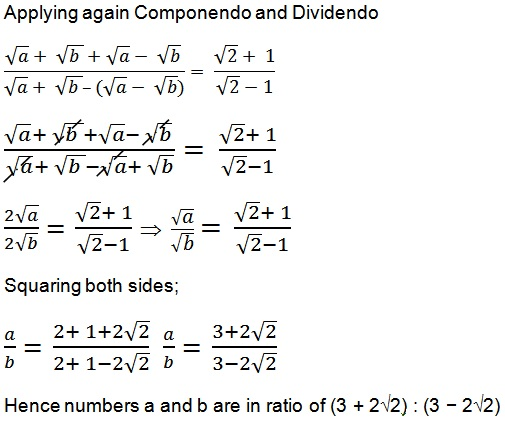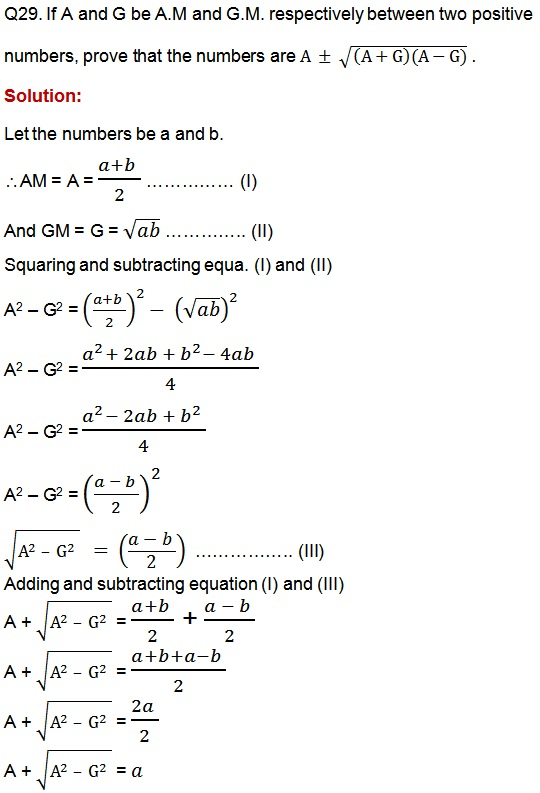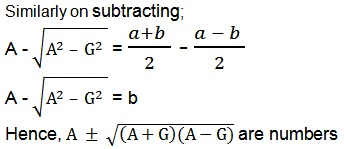Important Study materials for classes 06, 07, 08,09,10, 11 and 12. Like CBSE Notes, Notes for Science, Notes for maths, Notes for Social Science, Notes for Accountancy, Notes for Economics, Notes for political Science, Noes for History, Notes For Bussiness Study, Physical Educations, Sample Papers, Test Papers, Mock Test Papers, Support Materials and Books.Mathematics Class - 11th

NCERT Maths book for CBSE Students.

books

## Study Materials List:

##### Solutions ⇒ Class 11th ⇒ Mathematics
1. Sets
2. Relations and Functions
3. Trigonometric Functions
4. Principle Of Mathematical Induction
5. Complex Numbers and Quadratic Equations
6. Linear Inequalities
7. Permutations and Combinations
8. Binomial Theorem
9. Sequences and Series
10. Straight Lines
11. Conic Sections
12. Introduction to Three Dimensional Geometry
13. Limits and Derivatives
14. Mathematical Reasoning
15. Statistics
16. Probability

New Books